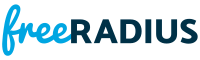eapcrypto.c File Reference
#include <stdio.h>
#include <stdlib.h>
#include "eap_types.h"
#include "eap_sim.h"Include dependency graph for eapcrypto.c:

Go to the source code of this file.

Functions

void eapsim_calculate_keys (struct eapsim_keys *ek)

void eapsim_dump_mk (struct eapsim_keys *ek)

Function Documentation

 void eapsim_calculate_keys ( struct eapsim_keys * ek )

Definition at line 37 of file eapcrypto.c.Here is the call graph for this function:Here is the caller graph for this function:

 void eapsim_dump_mk ( struct eapsim_keys * ek )

Definition at line 103 of file eapcrypto.c.Here is the caller graph for this function: